# Catch Maximum Sustainable Yield (cMSY)

Catch MSY (cMSY) is an assessment method for data-limited fisheries in cases where approximate catches are known preferably from the start of exploitation, but there are no indices of abundance or informative composition data. The approach does not require any additional information such as natural mortality. The method was published by Froese et al. (Fish and Fisheries 18(3): 506-526).

cMSY is a Monte Carlo production model for estimating fisheries reference points such as Maximum Sustainable Yield (MSY) from catch data, some input prior information about resilience and qualitative stock information.

Although there are several packages that can be used to run cMSY, the one by Dr Malcolm Haddon is supported here, because his cMSY version has been applied in several cases in Australia.

# Installation

This package is run from R as found on CRAN. R can be installed at https://cran.r-project.org/.

The recommended way to run R is using RStudio. After you have installed R, then install RStudio. It can be found at https://www.rstudio.com/products/rstudio/download/.

The supported version of cMSY is part of a set of stock assessment tools within Dr Haddon’s datalowSA package. Therefore one needs to first install datalowSA from within R.

Set up checking/installing packages functions

``````# Ordinary checking/installing function
Check_Install.packages <- function(pkg){
new.pkg <- pkg[!(pkg %in% installed.packages()[, "Package"])]
if (length(new.pkg))
install.packages(new.pkg,dependencies = TRUE) else print(paste0("'",pkg,"' has been installed already!"))
sapply(pkg, require, character.only = TRUE)
}

# github checking/installing function
Github_CheckInstall.packages <- function(github_pkg){
pkg <- tail(unlist(strsplit(github_pkg,"/")),1)
new.pkg <- pkg[!(pkg %in% installed.packages()[, "Package"])]
if (length(new.pkg))
devtools::install_github(github_pkg,build_vignettes=TRUE)
sapply(pkg, require, character.only = TRUE)
}``````

Installing packages from GitHub directly.

Check and install “devtools”

``Check_Install.packages("devtools")``
``##  "'devtools' has been installed already!"``
``## Loading required package: devtools``
``## Warning: package 'devtools' was built under R version 4.0.3``
``## Loading required package: usethis``
``````## devtools
##     TRUE``````

Check and install datalowSA from github

``````# Check if existed and if not, install the required package TMBhelper:
Github_CheckInstall.packages(github_pkg=github_pkg)``````
``## Loading required package: datalowSA``
``````## datalowSA
##      TRUE``````
``#devtools::install_github("https://github.com/haddonm/datalowSA",build_vignettes=TRUE)``

NB Once you have installed datalowSA, you don’t need to run the install.packages code chunk again. Luckily it will just give an error stating it won’t re-install if your version is up to date.

``library(datalowSA)``

It should be noted that there are also good vignettes for this package by typing browseVignettes(package = “datalowSA”). In that file for cMSY, Dr Haddon describes several steps of which we only show a few below with our example dataset.

An simulated example dataset has been created for the whole toolbox. Let us now read in these data. We assume you have saved the data to the same directory as your working directory.

``````Catches <- read.csv("Catches.csv")
# Read in the simulated annual catch data set
Catches <- Catches[-c(1:11),c(1,3)]
# We have removed the first 11 rows of data as these are all prefishery and zero,
# We have also removed the fleet column as there is only one fleet so is not needed
names(Catches) <- c("year","catch")
# this makes sure the headings are correct for later use
``````##    year     catch
## 12 1971   98.9982
## 13 1972  199.1610
## 14 1973  991.5380
## 15 1974  988.8430
## 16 1975 1978.3500
## 17 1976 1999.4000``````

Our dataset was created using Stock Synthesis based on an age-at-50%-maturity of roughly 10-11 years and a rate of natural mortality of 0.2 yr-1 for females and 0.25 yr-1 for males. The catch data are in metric tons.

### Fitting the model to our data

run_cMSY is the function that sets up and runs the cMSY model and simulations. Input data expected are a column of years and catches (as we have set up in Example). The model also needs some idea of the resilience of the population as a globals list which we can call glb, copying the example in datalowSA. The values of resilience can be ‘verylow’, ‘low’, ‘medium, or ’high’’ - with associated r ranges of (0.015 - 0.125), (0.1 - 0.6), (0.3 - 0.8), and (0.6 - 1.5). In the original code associated with the Martell and Froese (2013) paper the “medium”category is omitted. The last part of glb is the species name spsname. So lets create the list for glb which we could of course have read in.

Stock Synthesis, which was used to generate the simulated test dataset, does not directly compute r so we use a general assumption that \(r=2*Fmsy\) which means that r is about 0.3 which puts it into the medium range.

Create the resilience of the population as a globals list:

``````resilience <- "medium"
spsname <- "Codoid"
glb <- list(resilience = resilience,spsname = spsname)
print(glb)``````
``````## \$resilience
##  "medium"
##
## \$spsname
##  "Codoid"``````

## Running the model

Now that we have the data and inputs set up, the next is to run the model itself. Since it is a Monte Carlo approach, one needs to state how many simulations to undertake. One would normally run at least 20,000 simulations, even preferably more. Since the model runs quickly, we have set it at 40,000. If this is not set, then it defaults to 10,000.

There are several other settings, for which one could just use the defaults. The text describing these are taken directly from Dr Haddon’s vignette for cMSY:

• incB: the increments between the bounds of the initial biomass depletion levels (i.e Binitial/Bvirgin); defaults to 0.025, but have used 0.05 previously

• sigpR: the measure of process error added to the dynamics. If set to a very small value, 1e-06, the model will act as deterministic.

• multK: a multiplier for K to allow for stock biomasses to rise above K, defaults to 1.0, i.e. K is the upper limit.

• finaldepl: this allows the option of externally setting the final depletion where there have been major reductions in catch that have not been due to a reduction in the stock; defaults to NA, which sets the finaldepl to the pre-defined priors

• start_k: allows an option to alter the starting K values; for example in Orange Roughy, very large initial catches possibly make up a significant proportion of the initial biomass so multiplying by 60 or 100 will lead to ridiculous initial K values. A vector of two numbers is required. The default is NA, which means it will be c(maxcatch,60*maxcatch)

• start_r: allows for altering the default starting r values; for example in a species thought to be of resilience verylow there may be uncertainty over how low and one might want a range from say 0.01 - 0.3 instead of 0.015 - 0.125. The default is NA, which implies the default schedule of values in the code will be used. A vector of two numbers is required.

• initdepl: this allows the option of externally setting the initial depletion. This may be useful where there is evidence that the stock really has been unfished and is expected to be much closer to carrying capacity than the default setting of c(0.5, 0.975); defaults to NA, which sets the initdepl to the pre-defined priors

• maximumH: upper limit of harvest rate included in the constraints; defaults to 1.0, which implies no upper limit.

• Hyear: is the index to the year in which a range of harvest rates is to be used to constrain the acceptable trajectories.

Run model:

``````#library(datalowSA)
reps <- 40000
run1 <- run_cMSY(Catches,glb,n=reps) # this version uses all the defaults
summary <- summarycMSY(run1,Catches,final=TRUE)
names(summary)``````
``````##   "countcolour" "meanmsy"     "meanr"       "meanK"       "r"
##   "K"           "msy"         "pickC"       "years"       "parbound"
##  "fish"``````

From these you can see that the raw results are stored in run1. summarycMSY generates a list with key information that you will need to have a closer look at the results. The final command considers whether to use the first or last phase - TRUE is the default and that means using the final phase.

run1\$R1 contains the table of biomass trajectories, the identifier that denotes whether the individual trajectories within each r-K pair succeeded, and the r-K pairs that were trailed.

Let’s have a look at these results.

• plottrajectory: plots the predicted trajectories from the parameter combinations that have been accepted. Now here you can plot the trajectories that have succeeded using: +plotall: plots a set number of the r-K pairs, usually numbers between 7 and 15 are best. If plotall is TRUE then all trajectories are shown. +oneplot: if TRUE, then all the trajectories are provided in a single plot with median values in red. plotall settings are then irrelevant and uses the default of 7. +inparbound: the set of successful trajectories in the runs. In our case ru1\$parbound provides the range of values of r,K, initial and final depletion, and sigR.

### Using the defaults

Let’s look at the defaults first (OutDefault) and then narrow down to only the successful ones (OutSubsetNew). This figure gives 7 examples (because we set plotall=7 below) of the different r-K combinations (shown as the y-axis labels), illustrating those trajectories that were plausible. The number at the top of each plot is the predicted MSY for the given r-K pair shown next to the y-axis. The final plot is the catch history with the predicted average MSY as the blue line.

``````outDefault <- plottrajectory(inR1=run1\$R1,years=Catches\$year,
catch=Catches\$catch,inparbound=run1\$parbound,oneplot=FALSE,
scalar=1.0,plotout=TRUE,plotall=7) ``````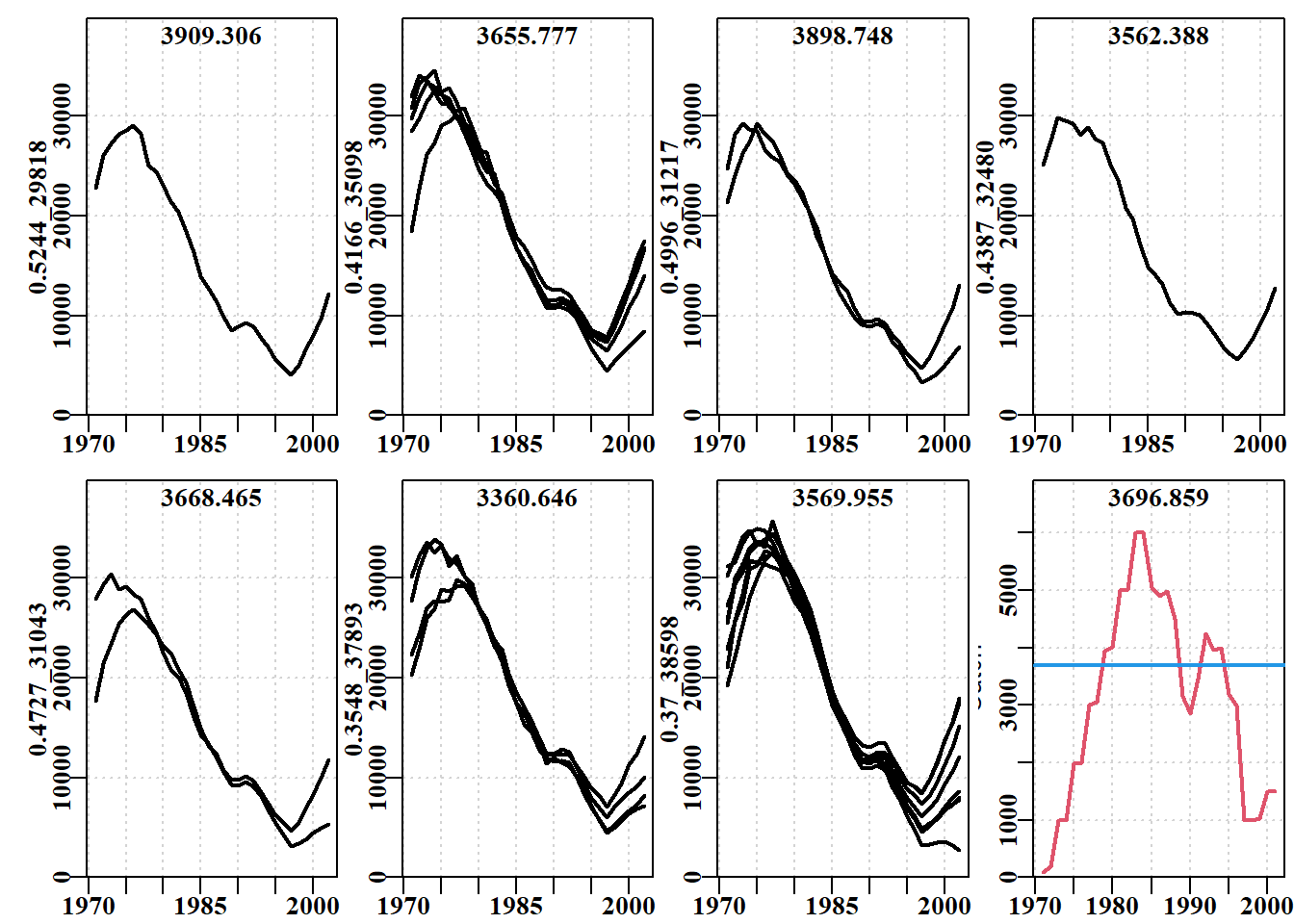``````outSubset <- plottrajectory(inR1=run1\$R1,years=Catches\$year,
catch=Catches\$catch,inparbound=run1\$parbound,oneplot=TRUE,
scalar=1.0,plotout=TRUE,plotall=7) ``````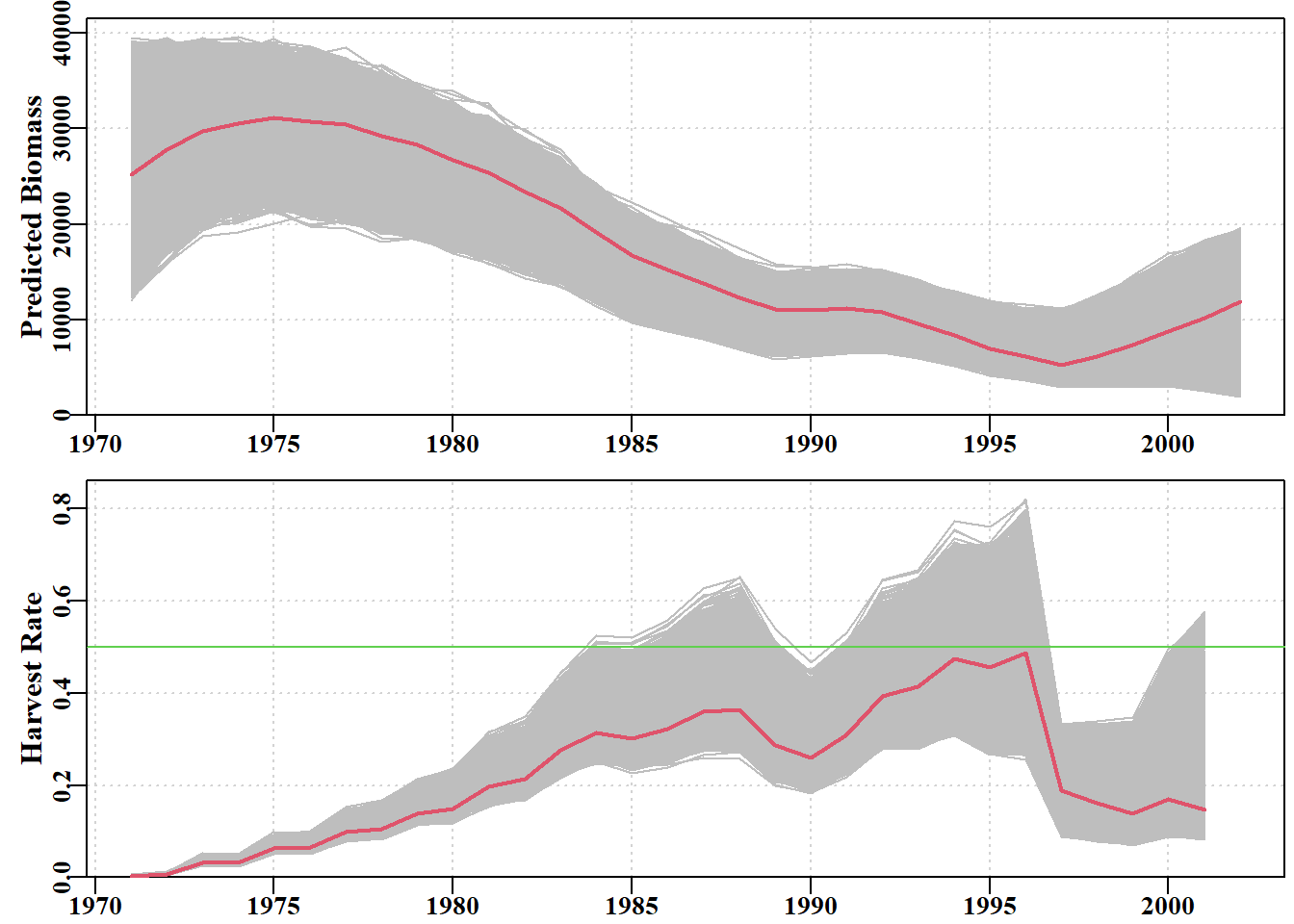However, one of the features of this dataset is that the catch data starts at the start of the fishery i.e. the resource is an unexploited stock at the start of the time series. So we can therefore change the initial depletion to close to 100% by setting it to being between 0.95 and 1.0.

``````runNew <- run_cMSY(Catches,glb,n=reps, initdepl=c(0.95,1.0))
summaryNew <- summarycMSY(runNew,Catches,final=TRUE)
outSubsetNew <- plottrajectory(runNew\$R1,
Catches\$year,Catches\$catch,runNew\$parbound,
oneplot=TRUE,scalar=1.0,plotout=TRUE,plotall=7) ``````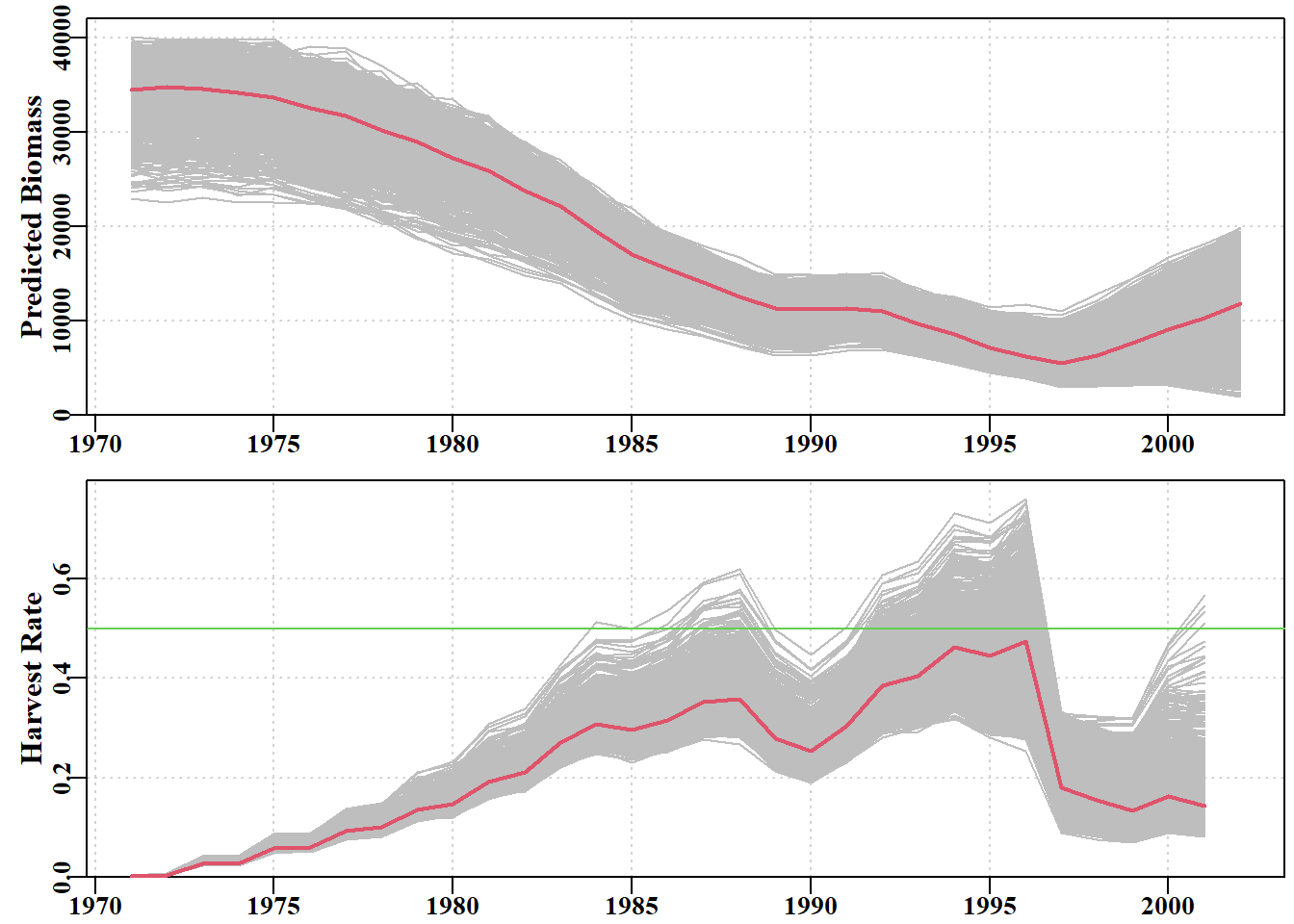Let’s then add a few more graphs. The first is useful in giving us information about the parameters themselves and the likely values for MSY. This command is plotcMSY6 which uses the summary results, the catch series and the species name.

plotCMSY produces a plot of the catch trajectory with the MSY and its 90% percentiles in the top left plot. The top middle plot shows the r and K combinations, and the top right shows the log-transformed version of this plot (i.e. ln(K) and ln(r)). In these plots the red dots depict failure, whereas the increasing intensity colours depict more combinations of initial depletion that succeeded for each r-K pair. The histograms at the bottom describe the distributions of the successful r and K values, and the resulting MSY distribution. The red lines are the median and the 90th percentile confidence intervals.

``print(plotcMSY6(summaryNew,Catches[,"catch"],label=glb\$spsname))``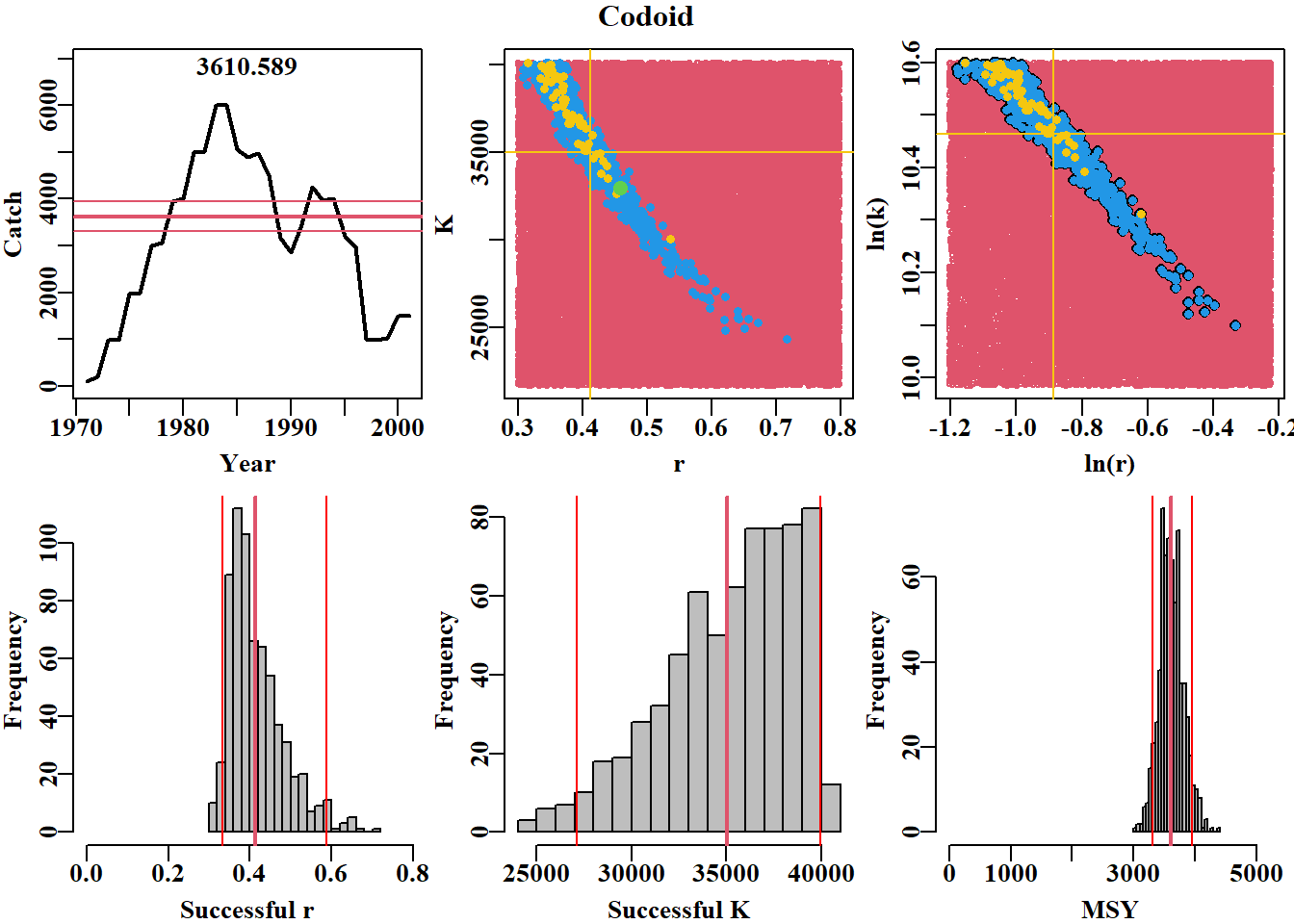``## NULL``

### Run with actual information

Let’s compare these results with the actual using the known biology of the species. For example the final depletion is 0.27.

``````runActual <- run_cMSY(Catches,glb,n=reps, initdepl=c(0.95,1.0), finaldepl = c(0.25,0.3), start_r = c(0.2,0.4))
summaryActual <- summarycMSY(runActual,Catches,final=TRUE)
outSubsetActual <- plottrajectory(runActual\$R1,
Catches\$year,Catches\$catch,runActual\$parbound,
oneplot=TRUE,scalar=1.0,plotout=TRUE,plotall=7) ``````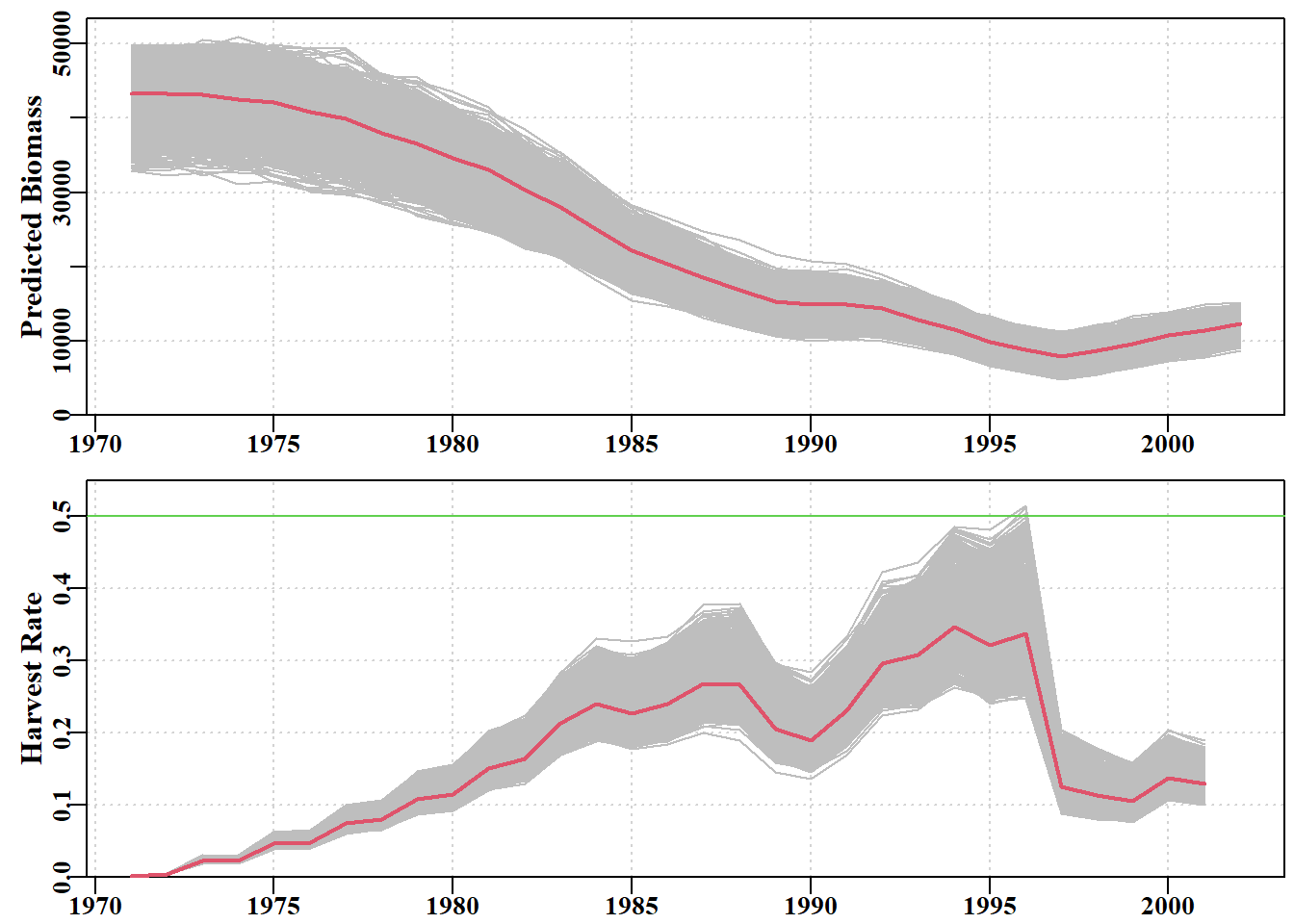``````print(plotcMSY6(summaryActual,Catches[,"catch"],
label=glb\$spsname))``````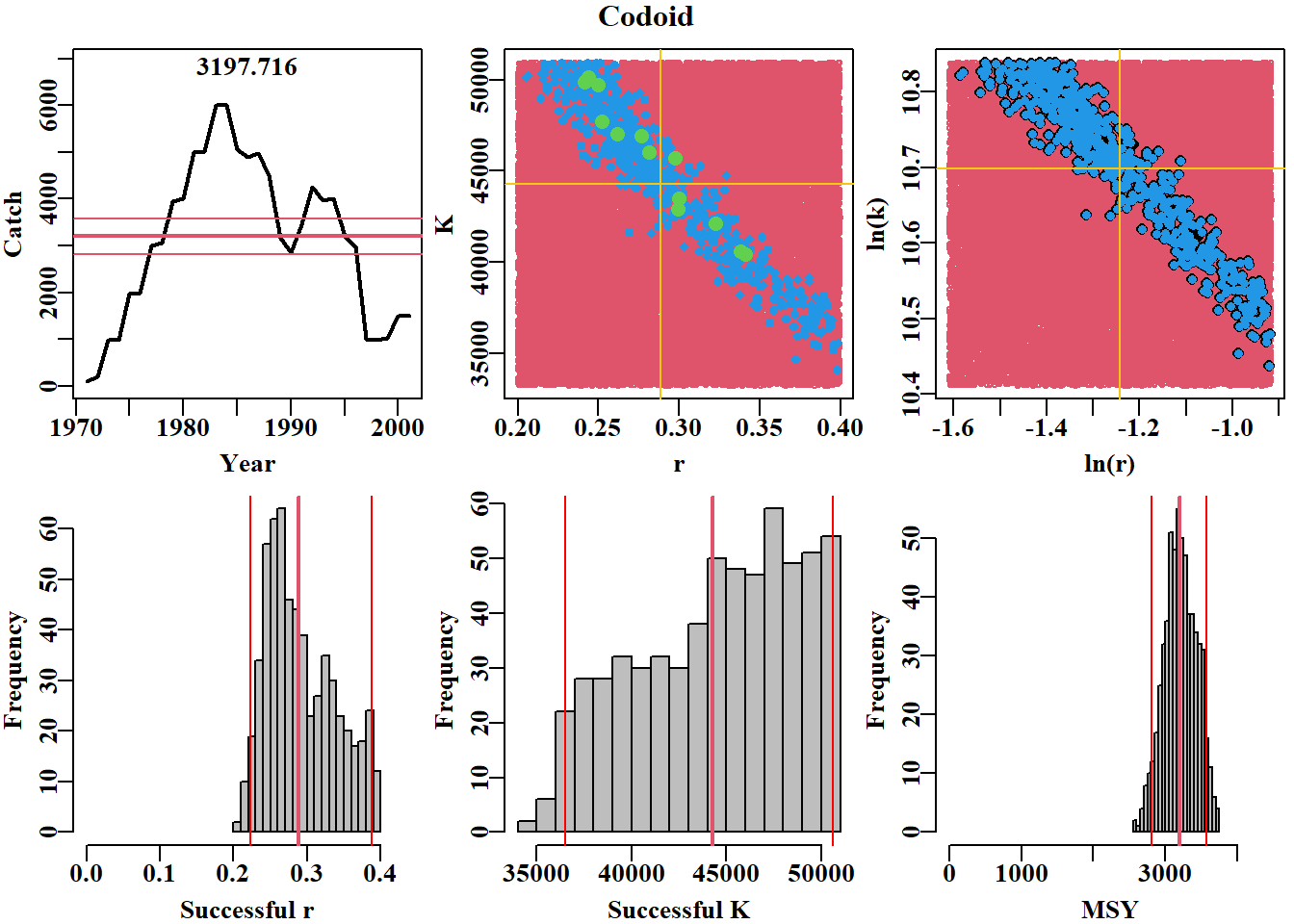``## NULL``

## Interpreting results in a management context

From the above graphs one can already get quite a lot of the required management information, but most importantly MSY and the harvest rate. However, the depletion is still not shown in these plotStock status relative to classic target (e.g. 0.4B0) and limit (0.2B0) reference point proxies.

• pulloutStats: summarises the results from your cMSY analysis (now runNew) and generates the mean, minimum, maximum and quantile for r, K, MSY and the final year’s depletion over accepted simulations. probabs are the quantile values. Here the default values are used. Several different kinds of graphs can be made from these data, but we leave that for you to take further.

• Different constant catch projections can be using the command plotconstC. The first is a constant catch of 0. The second is the maximum catch.

• gettraject extracts the final plausible biomass trajectories from runNew\$R1. Within this function, is an option projn that creates space for biomass trajectories so an answer of 5 means that the last 5 years of your projections will be NA until you run doproject. Let’s do a projection with the catches at the end of the series.

• Finally, cMSYphaseplot extracts the data to produce the classic phase plot. BMSY = 0.5B0 for the Schaefer model; the green spot is the start of the data series and red is the end. cMSYphaseplot also plots the expected harvest rate that should lead to MSY, called Ftarg and the limit reference point, Flim. In most proxy environments: points above the Flim line (or Ftarg</ depending on which management objectives are used) would be classified as over-fishing leading to a status of ‘depleting’ and points to the left of 0.2B0 would be over-fished or ‘depleted’. In addition, the function plots the catch history and the implied harvest rates just below the phase plot.

``````endYear <- as.numeric(max(Catches\$year))
meanCatch <- mean(Catches\$catch)
meanCatch``````
``##  3084.323``
``````lastCatch <- Catches\$catch[Catches\$year==endYear]
lastCatch``````
``##  1491.04``
``````#plausible trajectory stats
ans <- pulloutStats(runNew\$R1,
probabs=c(0.025,0.05,0.5,0.95,0.975))

#trajectories with the last years catch
traject <- gettraject(runNew\$R1,projn=5)
newtraj <- doproject(traject,constC=lastCatch)
trajdepl <- makedeplet(newtraj)
plotconstC(trajdepl,endyear=endYear,constC=lastCatch,limit=0.2,target=0.40)``````